#There are 366 different Starters of The Day, many to choose from. You will find below some starters on the topic of Fractions. A lesson starter does not have to be on the same topic as the main part of the lesson or the topic of the previous lesson. It is often very useful to revise or explore other concepts by using a starter based on a totally different area of Mathematics.

Main Page

### Fractions Starters: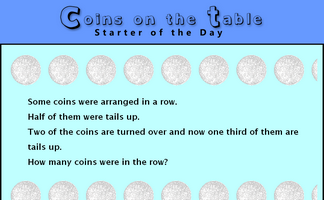A puzzle about the number of coins on a table given information about fractions of them.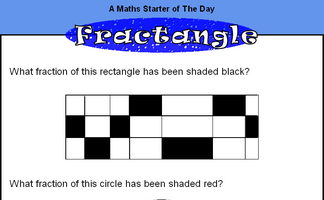Can you see what fractions of the shapes have been shaded? It is not as difficult as it first appears.Arrange the fractions and decimals in order from smallest to largest.Convert fractions to decimals, decimals to percentages and percentages to fractions.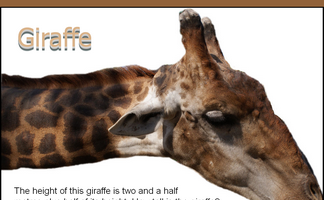The height of this giraffe is three and a half metres plus half of its height. How tall is the giraffe?Find the number which when added to the top (numerator) and bottom (denominator) of each fraction make it equivalent to one half.Five problems with a Halloween theme.Find fractions between two given values.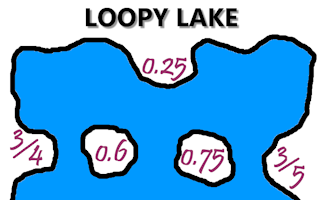Match the fractions to their decimal equivalents and join them with lines that do not cross.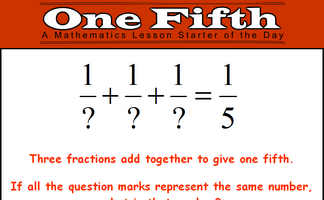Investigate three fractions which add together to give one fifth.Investigate three fractions which add together to give one ninth.Use your calculator to find which whole number divided by another whole number gives a recurring decimal.It is called Refreshing Revision because every time you refresh the page you get different revision questions.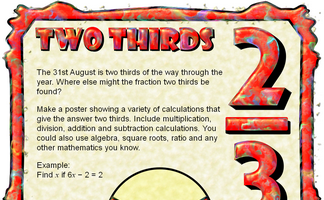Make a poster showing a variety of calculations that give the answer two thirds.

## Exercises#### Algebraic Fractions

A mixture of algebraic fraction calculations and simplifications.#### Boxed In Fractions

The classic dots and boxes two-player game with the addition of some fractions which determine your score.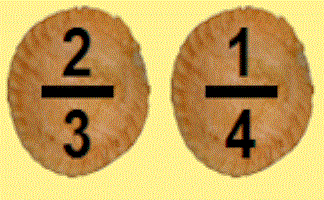#### Cheese and Onion Pies

Sort the fractions from smallest to largest.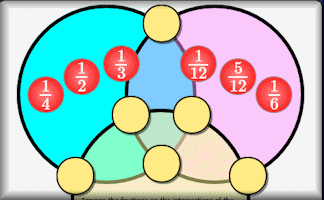#### Circumfraction

Quite a challenging number placing puzzle involving fractions.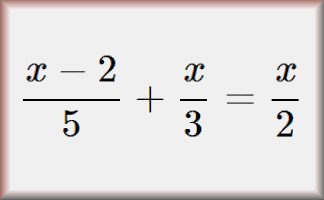#### Equations with Fractions

Practise solving linear equations that contain fractions in this multi-level exercise.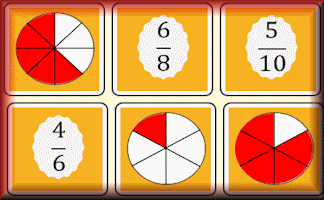#### Equivalent Fraction Pairs

The traditional pairs or Pelmanism game adapted to test knowledge of equivalent fractions.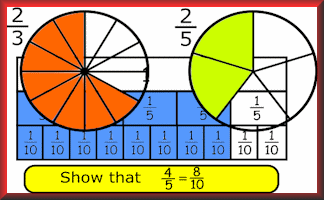#### Equivalent Fractions

Practise finding equivalent fractions numerically and in fraction diagrams.#### Express as a Fraction

Express one quantity as a fraction of another, where the fraction could be less than 1 or a mixed number.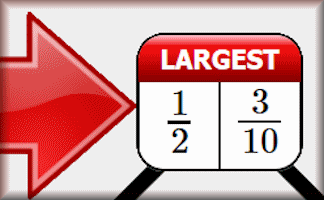#### Fickle Fractions

Compare pairs of fractions to identify the largest or smallest in order to move through the maze.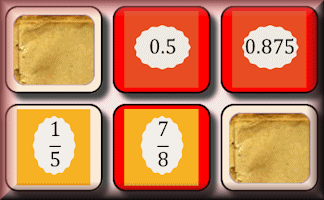#### Fraction Decimal Pairs

The traditional pairs or Pelmanism game adapted to test knowledge of simple fractions and their equivalent decimals.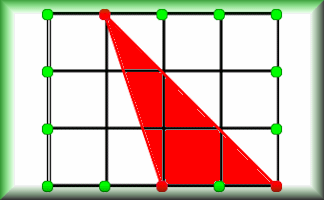#### Fraction Dissect

Draw lines to dissect the rectangles to make the given fractions.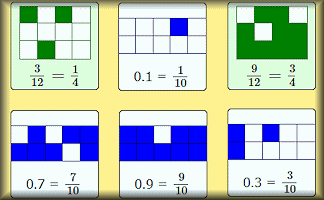#### Fraction Line

Arrange the fractions, shown in a variety of formats, in order from smallest to largest.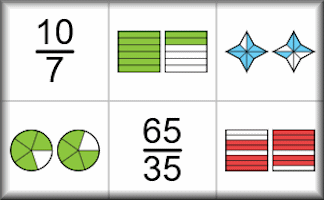#### Fraction Matcher

Match shapes and numbers in this fractions activity. Challenge yourself on many levels.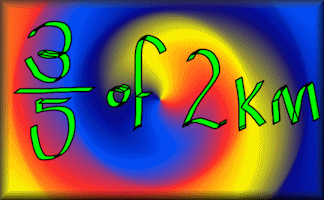#### Fraction of ...

Practise your ability to find a fraction of a given amount with this self marking exercise.#### Fraction Order

Arrange the fractions and decimals in order from smallest to largest.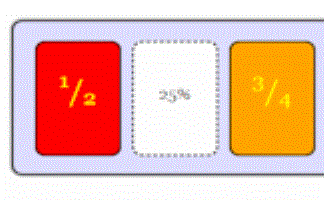#### Fraction Percentage

Match the fraction with the equivalent percentage. A drag and drop self marking exercise.#### Fraction Percentage Pairs

The traditional pairs or Pelmanism game adapted to test knowledge of simple fractions and their equivalent percentages.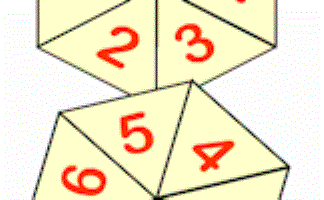#### Fraction Spinners

Know the properties of the fractions randomly produced by the two spinners.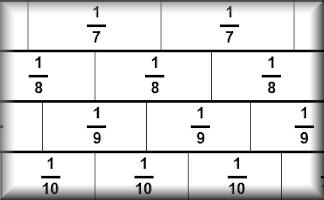#### Fraction Wall

The traditional fraction wall diagram showing the relationship between simple fractions.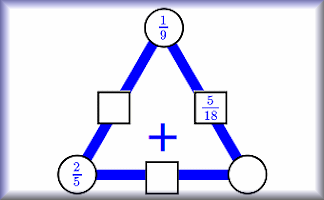#### Fractionagons

Calculate the missing fractions in these partly completed arithmagon puzzles.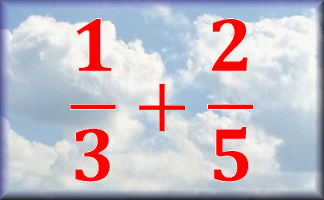#### Fractions

A series of self-marking exercises on adding, subtracting, multiplying and dividing fractions.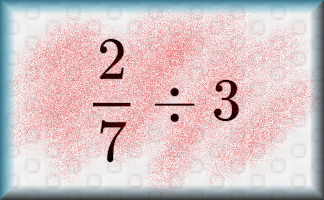#### Fractions by Wholes

An exercise on multiplying and dividing proper fractions and mixed numbers by whole numbers.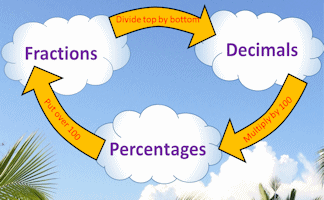#### Fractions Decimals Percentages

Revise the methods for converting fractions to decimals and percentages.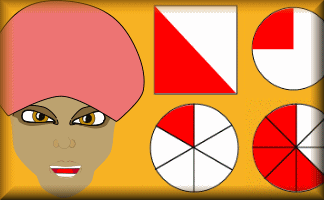#### Fractions Kim's Game

A memory game to be projected to help the whole class recognise basic fractions.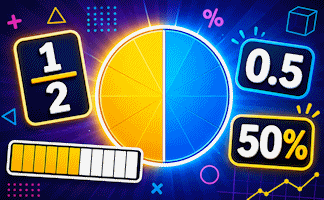#### Fractions, Decimals, Percentages

An exercise on converting fractions to decimals, decimals to percentages and percentages to fractions.#### How old was Diophantus?

An ancient riddle which can be answered by solving an equation containing fractions.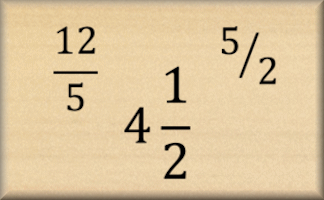#### Improper Fractions

A self-marking online exercise on converting improper fractions to mixed numbers and vice versa.#### Inequalities

Check that you know what inequality signs mean and how they are used to compare two quantities. Includes negative numbers, decimals, fractions and metric measures.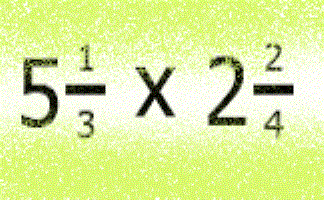#### Mixed Numbers

A self marking quiz about the application of the four operations to mixed numbers.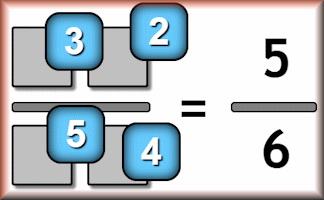A set of ten puzzles requiring you to arrange the given digits to make an equivalent fraction.#### Particular Pipes

Construct the pipes using a set number of pieces with lengths given as fractions, decimals or percentages.#### Ratios vs Fractions

Relate the language of ratios and the associated calculations to the arithmetic of fractions.#### Recurring Decimals

Change recurring decimals into their corresponding fractions and vica versa.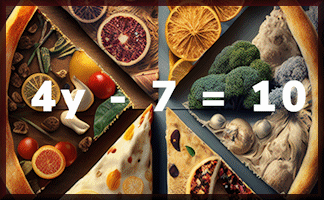#### Solve To Find Fractions

Find the value of the unknown in each of these linear equations. All of the answers are fractions#### Vinculum

A challenge to find fractions which are larger than the previous fraction but less than one.

### Search

The activity you are looking for may have been classified in a different way from the way you were expecting. You can search the whole of Transum Maths by using the box below.

Have today's Starter of the Day as your default homepage. Copy the URL below then select
Tools > Internet Options (Internet Explorer) then paste the URL into the homepage field.

Set as your homepage (if you are using Internet Explorer)

Do you have any comments? It is always useful to receive feedback and helps make this free resource even more useful for those learning Mathematics anywhere in the world. Click here to enter your comments.For All: